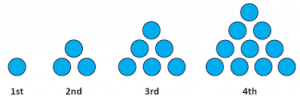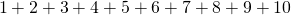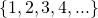# Arithmetic Series: Development

On the page Arithmetic Sequences: Introduction we considered the triangle numbers.To find out how many stones are needed to make the 4th shape, we have to calculate the following sum:Now since there are not so many terms here, it is quite a straightforward sum. There are 10 stones in the fourth shape.

Now to figure out how many stones are required to make the 10th shape, we need to calculate:Notice that the terms in this sum are the first ten terms of a very simple arithmetic sequence:We come now to a new definition:

 A series is the sum of a sequence, often called a partial sum, as sequences can be infinitely long.

There is a lovely formula for calculating the sum of an arithmetic progression. It uses a common trick, which is to add something and then to take it away later – in other words ‘adding zero’.

Let’s add together 1 + 2 + 3 + 4 + 5 as an example. The diagram below shows these numbers as rows in a triangle form. Let’s see how how the ‘trick’ works, and how it helps get the answer to the sum:

 Notice that the base of the rectangle is made up by the bottom row of the original triangle, plus the top row of the new triangle.

Now we can use the same method to calculate the number of stones in the 10th shape. Let’s answer these questions about the 10th shape:

1. How many rows are there?  10 rows.
2. What is the base of the rectangle? Bottom row plus top row = 10 + 1 = 11.
3. Number of stones in the rectangle:.
4. Number of stones in the rectangle divided by two:.

Therefore the number of stones in the 10th shape is 55.

We can use this method to find out how many stones are in any of the shapes.

How many stones do you need to make the 100th shape, should you decide to make it?

# Impressive Kid!

A mathematician named Karl Frederich Gauss has been called ‘the prince of mathematics’ – due to his many contributions to mathematics. While still a primary school child, he impressed his teachers by calculating that. His teacher had given the class the task, expecting it to take some time. But with Gauss in the room, the task was over in seconds! The website www.nrich.maths.org has a nicely written article on this story!

# Extend to other arithmetic sequences:

What is nice about this ‘create another, turn it upside-down’ technique is that it works for any arithmetic progression. The infomation to look for is the base of the rectangle – made up of the first and the last terms of the sum; and the height of the rectangle – which is the number of terms in the sequence. Let’s try out this technique with the following sequence:Calculate the sum of the first 8 terms.

On this applet, build the rectangle by drawing the first 8 terms of the sequence, and then draw them in reverse.

• What are the dimensions of the resulting rectangle?
• What is the sum of all the first 8 terms of this sequence?
• Why do the descending rows match the ascending rows?
• Why will they always do this for an arithmetic sequence?

# Write the process as a formula

When faced with a calculation, it is often helpful to give it a name. Our first step in the process of writing a formula is to give the ‘thing we don’t know’ a name. Let’s call the sum of the first 8 terms of this sequence1. Name the total number of stones2. Imagine a second triangle with the same number of stones – now you have.
3. By inverting the second triangle, you create a rectangle. The base of the rectangle is made up ofand. The base.
4. The height of the rectangle is the number of terms being added – in this case,. We usually refer to the number of terms with the letter.
5. The number of stones,is equal to half of the number of stones in the rectangle.

In other words,Our formula is therefore,Which can be remembered as ‘first term plus last term, multiply by the number of terms, divided by 2’.

# A little more algebra

Notice, in our formula, we see the value ‘‘.

Now, in the last example where, we calculatedusing our formula,.

We can pick out the termfrom the formula, and simply replace it with its calculation:. Then simplify. We have:This is often written as## Short Comprehension Exercises

The two formula can be used interchangeably, however you can save yourself one line of work if you use the formula that requires the information you have. That is, the formulais most useful when you have the first term, the last term, and the number of terms. The formulais most useful when you have the first term, the common difference, and the number of terms.

Using either of these formulae, calculate:

1. The sum of the first 12 positive multiples of 5. (That is, 5, 10, 15 … 55, 60).
2. The sum of the first 20 terms of the arithmetic sequence beginning 9, 16, 23 …
3. The sum of the first 41 terms of the arithmetic sequence beginning 80, 76, 72 …

## More Difficult Question

The sum of an arithmetic progression is given by the formula. Find the fifth term of the sequence.

CEMC courseware: Lesson 5 arithmetic series

I’m done, take me back to the Sequences and Series Menu!